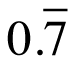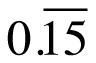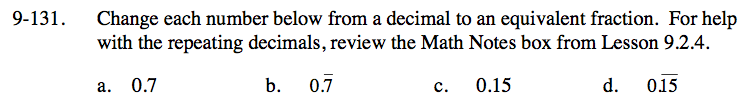Home > CC3 > Chapter 9 > Lesson 9.2.5 > Problem9-131

9-131.
1. Change each number below from a decimal to an equivalent fraction. For help with the repeating decimals, review the Math Notes box from Lesson 9.2.4. Homework Help ✎

1. 0.7

2.3. 0.15

4.This decimal can be read as seven-tenths. What fraction is also read that way?

$\frac{7}{10}$

There is only one repeating digit, so you put it over 9. Don't forget to reduce if you can!

$\frac{7}{9}$

See the hint for part (a).

See the hint for part (b).